# Using Tables to Compare Ratios Online Quiz

#### Complete Python Prime Pack

9 Courses     2 eBooks

#### Artificial Intelligence & Machine Learning Prime Pack

6 Courses     1 eBooks

#### Java Prime Pack

9 Courses     2 eBooks

Following quiz provides Multiple Choice Questions (MCQs) related to Using Tables to Compare Ratios. You will have to read all the given answers and click over the correct answer. If you are not sure about the answer then you can check the answer using Show Answer button. You can use Next Quiz button to check new set of questions in the quiz.Q 1 - Use tables to compare the pair of ratios; 2:5; 4:9

### ExplanationStep 1:

The ratios and their equivalent ratios are given in tables above.

Step 2:

Comparing the fractions, $\frac{4}{9}$ is > $\frac{4}{10}$

Step 3:

So, $4:9 > 2:5$

Q 2 - Use tables to compare the pair of ratios; 2:5; 3:8

### Explanation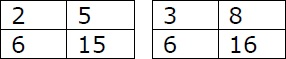Step 1:

The ratios and their equivalent ratios are given in tables above.

Step 2:

Comparing the fractions, $\frac{6}{15}$ is > $\frac{6}{16}$

Step 3:

So, $2:5 > 3:8$

Q 3 - Use tables to compare the pair of ratios; 2:5; 5:6

### ExplanationStep 1:

The ratios and their equivalent ratios are given in tables above.

Step 2:

Comparing the fractions, $\frac{10}{12}$ is > $\frac{10}{25}$

Step 3:

So, $5:6 > 2:5$

Q 4 - Use tables to compare the pair of ratios; 2:5; 5:7

### ExplanationStep 1:

The ratios and their equivalent ratios are given in tables above.

Step 2:

Comparing the fractions, $\frac{10}{14}$ is > $\frac{10}{25}$

Step 3:

So, $2:5 < 5:7$

Q 5 - Use tables to compare the pair of ratios; 2:5; 5:9

### Explanation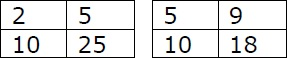Step 1:

The ratios and their equivalent ratios are given in tables above.

Step 2:

Comparing the fractions, $\frac{10}{18}$ is > $\frac{10}{25}$

Step 3:

So, $2:5 < 5.9$

Q 6 - Use tables to compare the pair of ratios; 2:3; 3:4

### Explanation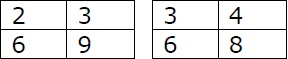Step 1:

The ratios and their equivalent ratios are given in tables above.

Step 2:

Comparing the fractions, $\frac{6}{8}$ is > $\frac{6}{9}$

Step 3:

So, $2:3 < 3:4$

Q 7 - Use tables to compare the pair of ratios; 2:3; 3:5

### Explanation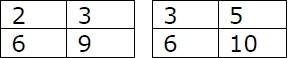Step 1:

The ratios and their equivalent ratios are given in tables above.

Step 2:

Comparing the fractions, $\frac{6}{9}$ is > $\frac{6}{10}$

Step 3:

So, $2:3 > 3:5$

Q 8 - Use tables to compare the pair of ratios; 2:3; 3:7

### ExplanationStep 1:

The ratios and their equivalent ratios are given in tables above.

Step 2:

Comparing the fractions, $\frac{6}{9}$ is > $\frac{6}{14}$

Step 3:

So, $2:3 > 3:7$

Q 9 - Use tables to compare the pair of ratios; 2:3; 4:5

### Explanation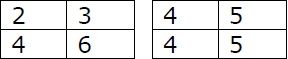Step 1:

The ratios and their equivalent ratios are given in tables above.

Step 2:

Comparing the fractions, $\frac{4}{5}$ is > $\frac{4}{6}$

Step 3:

So, $2:3 < 4:5$

Q 10 - Use tables to compare the pair of ratios; 2:3; 4:7

### Explanation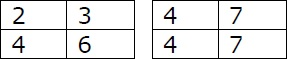Step 1:

The ratios and their equivalent ratios are given in tables above.

Step 2:

Comparing the fractions, $\frac{4}{6}$ is > $\frac{4}{7}$

Step 3:

So, $2:3 > 4:7$

using_tables_compare_ratios.htm